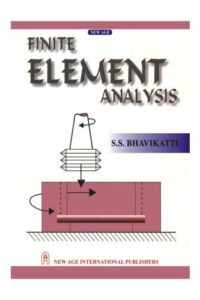اسم المؤلف
S. S. BHAVIKATTI
التاريخ
6 يونيو 2018
المشاهدات
43
التقييمLoading...

Finite Element Analysis
S. S. BHAVIKATTI
Sri Dhirmasthal Manjunatheshwara College of Engineering ami
(Formerly).Professor and Head Department of Civil Engineering
Karnataka Regional Engineering College, Surathkal
Preface v
Acknowledgements vi
1. Introduction 1
1.1 General 1
1.2 General Description of the Method 1
1.3 Brief Explanation of FEA for a Stress Analysis Problem 2
1.4 Finite Element Method vs Classical Method 4
1.5 FEM vs FDM 5
1.6 A Brief History of FEM 6
1.7 Need for Studying FEM 6
1.8 Warning to FEA Package Users 7
Questions 7
References 7
2. Basic Equations in Elasticity 9
2.1 Introduction 9
2.2 Stresses in a Typical Element 9
2.3 Equations of Equilibrium 12
2.4 Strains 14
2.5 Strain Displacement Equations 14
2.6 Linear Constitutive Law 15
Questions 20
3. Matrix Displacement Formulation 21
3.1 Introduction 21
3.2 Matrix Displacement Equations 21
3.3 Solution of Matrix Displacement Equations 28
3.4 Techniques of Saving Computer Memory Requirements 30
Questions 32viii Contents
4. Element Shapes, Nodes, Nodal Unknowns and Coordinate Systems 33
4.1 Introduction 33
4.2 Element Shapes 33
4.3 Nodes 38
4.4 Nodal Unknowns 39
4.5 Coordinate Systems 40
Questions 53
5. Shape Functions 55
5.1 Introduction 55
5.2 Polynomial Shape Functions 56
5.3 Convergence Requirements of Shape Functions 59
5.4 Derivation of Shape Functions Using Polynomials 61
5.5 Finding Shape Functions Using Lagrange Polynomials 82
5.6 Shape Functions for Serendipity Family Elements 89
5.7 Hermite Polynomials as Shape Functions 95
5.8 Construction of Shape Functions by Degrading Technique 98
Questions 102
6. Strain Displacement Matrix 104
6.1 Introduction 104
6.2 Strain—Displacement Matrix for Bar Element 104
6.3 Strain Displacement Matrix for CST Element 105
6.4 Strain Displacement Relation for Beam Element 107
Questions 108
7. Assembling Stiffness Equation—Direct Approach 110
7.1 Introduction 110
7.2 Element Stiffness Matrix for CST Element by Direct Approach 110
7.3 Nodal Loads by Direct Approach 114
Questions 117
8. Assembling Stiffness Equation—Galerkin’s Method, 118
Virtual Work Method
8.1 Introduction 118
8.2 Galerkin’s Method 118
8.3 Galerkin’s Method Applied to Elasticity Problems 119
Questions 127Contents ix
9. Assembling Stiffness Equation—Variational Method 128
9.1 Introduction 128
9.2 General Variational Method in Elasticity Problems 128
9.3 Potential Energy in Elastic Bodies 134
9.4 Principles of Minimum Potential Energy 136
9.5 Rayleigh—Ritz Method 140
9.6 Variational Formulation in Finite Element Analysis 150
Questions 153
10. Discritization of a Structure 154
10.1 Introduction 154
10.2 Nodes as Discontinuities 154
10.3 Refining Mesh 156
10.4 Use of Symmetry 157
10.5 Finite Representation of Infinite Bodies 157
10.6 Element Aspect Ratio 158
10.7 Higher Order Element vs Mesh Refinement 159
10.8 Numbering System to Reduce Band Width 159
Questions 160
11. Finite Element Analysis—Bars and Trusses 161
11.1 Introduction 161
11.2 Tension Bars/Columns 161
11.3 Two Dimensional Trusses (Plane Trusses) 180
11.4 Three Dimensional Trusses (Space Trusses) 197
Questions 201
12. Finite Element Analysis—Plane Stress and Plane Strain Problems 204
12.1 Introduction 204
12.2 General Procedure when CST Elements are Used 204
12.3 Use of Higher Order Elements 216
Questions 217
13. Isoparametric Formulation 219
13.1 Introduction 219
13.2 Coordinate Transformation 221
13.3 Basic Theorems of Isoparametric Concept 222
13.4 Uniqueness of Mapping 223
13.5 Isoparametric, Superparametric and Subparametric Elements 224x Contents
13.6 Assembling Stiffness Matrix 225
13.7 Numerical Integration 230
13.8 Numerical Examples 232
Questions 240
References 241
14. Analysis of Beams and Rigid Frames 242
14.1 Introduction 242
14.2 Beam Analysis Using two Noded Elements 242
14.3 Analysis of Rigid Plane Frame Using 2 Noded Beam Elements 259
14.4 A Three Dimensional Rigid Frame Element 266
14.5 Timoshenko Beam Element 269
Questions 278
References 279
15. Bending of Thin Plates 280
15.1 Introduction 280
15.2 Basic Relations in Thin Plate Theory 281
15.3 Displacement Models for Plate Analysis 282
15.4 Rectangular Plate Element with 12 Degrees of Freedom 284
15.5 Rectangular Plate Element with 16 Degrees of Freedom 289
15.6 Mindlin’s Plate Element 292
Questions 299
References 299
16. Analysis of Shells 301
16.1 Introduction 301
16.2 Force on Shell Element 301
16.3 Finite Element for Shell Analysis 302
16.4 Finite Element Formulation Using Four Noded
Questions 317
References 317
17. Nonlinear Analysis 318
17.1 Introduction 318
17.2 Nonlinear Problems 318
17.3 Analysis of Material Nonlinear Problems 320
17.4 Analysis of Geometric Nonlinear Problems 325Contents xi
17.5 Analysis of Both Material and Geometric Nonlinear Problems 328
Questions 328
References 328
18. Standard Packages and Their Features 329
18.1 Introduction 329
18.2 Commercially Available Standard Packages 329
18.3 Structure of a Finite Element Analysis Program 330
18.4 Pre and Post Processors 331
18.5 Desirable Features of FEA Packages 333
Questions 333
كلمة سر فك الضغط : books-world.net